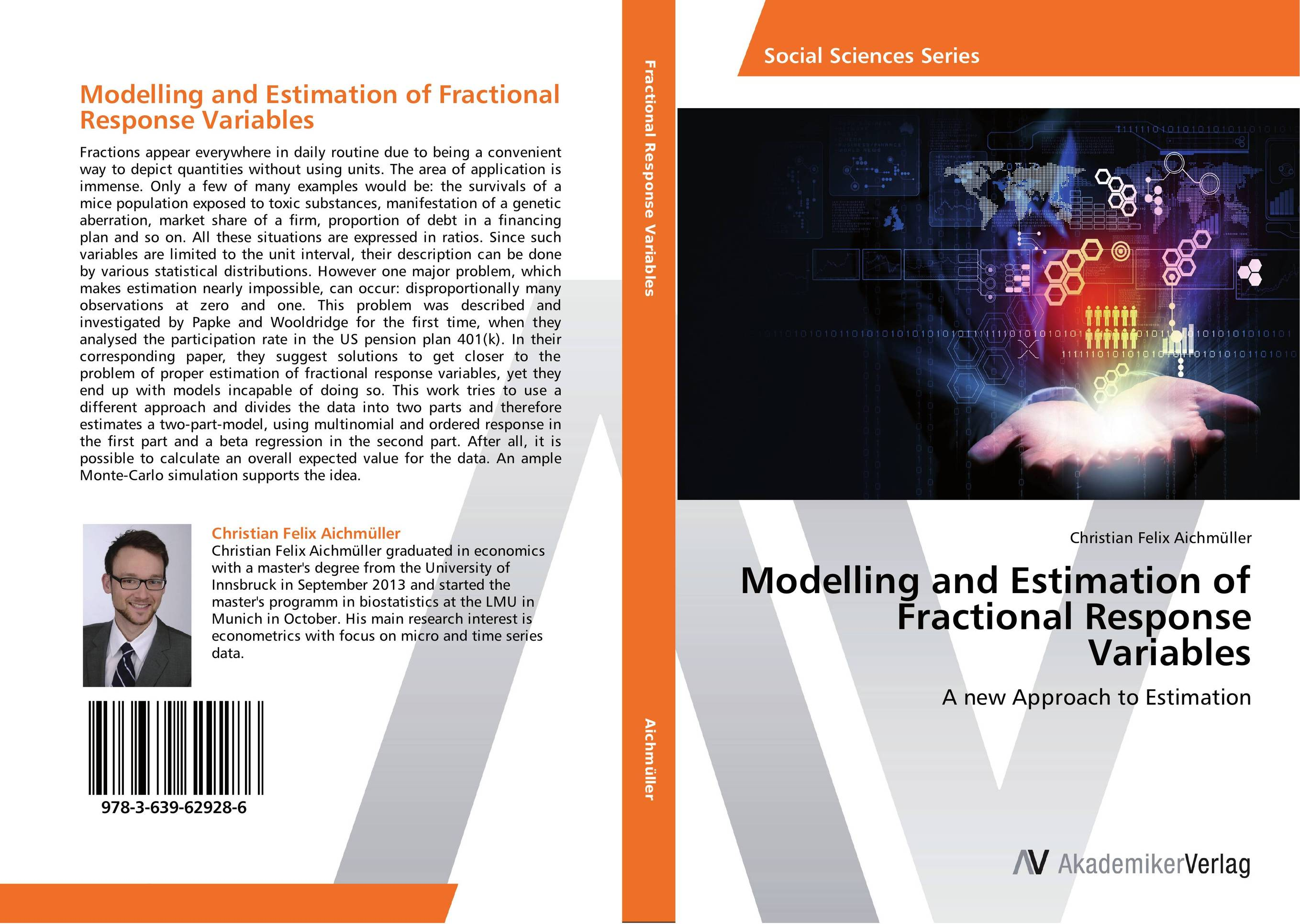# Modelling and Estimation of Fractional Response Variables

###### Аннотация:
Fractions appear everywhere in daily routine due to being a convenient way to depict quantities without using units. The area of application is immense. Only a few of many examples would be: the survivals of a mice population exposed to toxic substances, manifestation of a genetic aberration, market share of a firm, proportion of debt in a financing plan and so on. All these situations are expressed in ratios. Since such variables are limited to the unit interval, their description can be done by various statistical distributions. However one major problem, which makes estimation nearly impossible, can occur: disproportionally many observations at zero and one. This problem was described and investigated by Papke and Wooldridge for the first time, when they analysed the participation rate in the US pension plan 401(k). In their corresponding paper, they suggest solutions to get closer to the problem of proper estimation of fractional response variables, yet they end up with models incapable of doing so. This work tries to use a different approach and divides the data into two parts and therefore estimates a two-part-model, using multinomial and ordered response in the first part and a beta regression in the second part. After all, it is possible to calculate an overall expected value for the data. An ample Monte-Carlo simulation supports the idea.
###### Издания произведения:9783639629286

### Рекомендуем

#### Комментарии

Пока нет ни одного комментария
Вы должны войти для того что бы оставлять комментарии